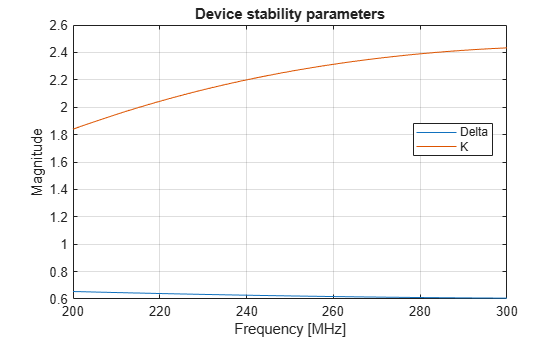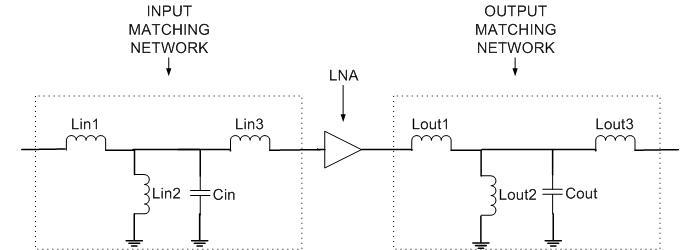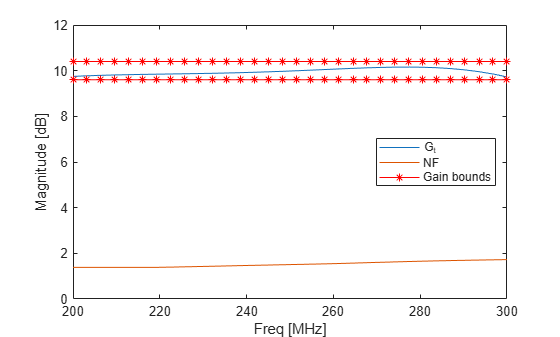# Designing Broadband Matching Networks (Part 2: Amplifier)

This example shows how to design broadband matching networks for a low noise amplifier (LNA). In an RF receiver front end, the LNA is commonly found immediately after the antenna or after the first bandpass filter that follows the antenna. Its position in the receiver chain ensures that it deals with weak signals that have significant noise content. As a result the LNA has to not only provide amplification to such signals but also minimize its own noise footprint on the amplified signal. In this example you will design an LNA to achieve the target gain and noise figure specifications over a specified bandwidth, using lumped LC elements. A direct-search based approach is used to arrive at the optimum element values in the input and output matching network.Figure 1: Impedance matching of an amplifier

### Set Design Parameters

The design specifications are as follows

• Amplifier is an LNA amplifier

• Center Frequency = 250 MHz

• Bandwidth = 100 MHz

• Transducer Gain greater than or equal to 10 dB

• Noise Figure less than or equal to 2.0 dB

• Operating between 50-Ohm terminations

### Specify Bandwidth, Center Frequency, Noise Figure, and Impedance

You are building the matching network for an LNA with a bandpass response, so specify the bandwidth of match, center frequency, gain, and noise figure targets.

```BW = 100e6; % Bandwidth of matching network (Hz) fc = 250e6; % Center frequency (Hz) Gt_target = 10; % Transducer gain target (dB) NFtarget = 2; % Max noise figure target (dB)```

Here you specify the source impedance, reference impedance, and the load impedance.

```Zs = 50; % Source impedance (Ohm) Z0 = 50; % Reference impedance (Ohm) Zl = 50; % Load impedance (Ohm)```

### Create Amplifier Object and Perform Analysis

Use the `read` method to create an amplifier object using data from the file `lnadata.s2p`.

`Unmatched_Amp = read(rfckt.amplifier,'lnadata.s2p'); % Create amplifier object`

Define the number of frequency points to use for analysis and set up the frequency vector.

```Npts = 32; % No. of analysis frequency points fLower = fc - (BW/2); % Lower band edge fUpper = fc + (BW/2); % Upper band edge freq = linspace(fLower,fUpper,Npts); % Frequency array for analysis w = 2*pi*freq; % Frequency (radians/sec)```

Use the `analyze` method to perform frequency-domain analysis at the frequency points in the vector freq.

`analyze(Unmatched_Amp,freq,Zl,Zs,Z0); % Analyze unmatched amplifier`

### Examine Stability, Power Gain, and Noise Figure

The LNA must operate in a stable region, so our first step is to plot `Delta` and `K` for the transistor being used. Use the `plot` method of the `rfckt` object to plot `Delta` and `K` as a function of frequency to see if the transistor is stable.

```figure plot(Unmatched_Amp,'Delta','mag') hold all plot(Unmatched_Amp,'K') title('Device stability parameters') hold off grid on```As the plot shows, $K>1$ and $\Delta <1$ for all frequencies in the bandwidth of interest. This means that the device is unconditionally stable. It is also important to view the power gain and noise figure behavior across the same bandwidth. Together with the stability information this data allows you to determine if the gain and noise figure targets can be met.

`plot(Unmatched_Amp,'Ga','Gt','dB')`This plot, shows the power gain across the 100-MHz bandwidth. It indicates that the transducer gain varies linearly between 5.5 dB to about 3.1 dB and achieves only 4.3 dB at band center. It also suggests there is sufficient headroom between the transducer gain `Gt` and the available gain Ga to achieve our target `Gt` of 10 dB.

```plot(Unmatched_Amp,'Fmin','NF','dB') axis([200 300 0 2]) legend('Location','NorthEast')```This plot shows the variation of the noise figure with frequency. The unmatched amplifier clearly meets the target noise figure requirement. However this would change once the input and output matching networks are included. Most likely, the noise figure of the LNA would exceed the requirement.

The region of operation is between 200 and 300 MHz, so you choose a bandpass topology for the matching networks which is shown here,Figure 2: Matching network topology

The topology chosen, as seen in Figure 2, is a direct-coupled prototype bandpass network of parallel resonator type with top coupling , that is initially tuned to the geometric mean frequency with respect to the bandwidth of operation.

```N_input = 3; % Order of input matching network N_output = 3; % Order of output matching network wU = 2*pi*fUpper; % Upper band edge wL = 2*pi*fLower; % Lower band edge w0 = sqrt(wL*wU); % Geometric mean```

For the initial design all the inductors are assigned the same value on the basis of the first series inductor. As mentioned in , choose the prototype value to be unity and use standard impedance and frequency transformations to obtain denormalized values . The value for the capacitor in the parallel trap is set using this inductor value to make it resonate at the geometric mean frequency. Please note that there are many ways of designing the initial matching network. This example shows one possible approach.

```LvaluesIn = (Zs/(wU-wL))*ones(N_input,1); % Series and shunt L's [H] CvaluesIn = 1 / ( (w0^2)*LvaluesIn(2)); % Shunt C [F]```

### Form Complete Circuit with Matching Networks and the Amplifier

Use either the `rfckt.seriesrlc` or `rfckt.shuntrlc` constructor to build each branch of the matching network. Then, form the matching network from these individual branches by creating an `rfckt.cascade` object. The output matching network for this example is the same as the input matching network.

```LC_InitialIn = [LvaluesIn;CvaluesIn]; LvaluesOut = LvaluesIn; CvaluesOut = CvaluesIn; LC_InitialOut = [LvaluesOut;CvaluesOut]; InputMatchingNW = rfckt.cascade('Ckts', ... {rfckt.seriesrlc('L',LvaluesIn(1)), ... rfckt.shuntrlc('C',CvaluesIn,'L',LvaluesIn(2)), ... rfckt.seriesrlc('L',LvaluesIn(3))}); OutputMatchingNW = rfckt.cascade('Ckts', ... {rfckt.seriesrlc('L',LvaluesOut(1)), ... rfckt.shuntrlc('C',CvaluesOut,'L',LvaluesOut(2)), ... rfckt.seriesrlc('L',LvaluesOut(3))});```

Put together the LNA network consisting of matching networks and amplifier by creating an `rfckt.cascade` object as shown in previous section.

```Matched_Amp = rfckt.cascade('Ckts', ... {InputMatchingNW,Unmatched_Amp,OutputMatchingNW});```

### Optimize Input & Output Matching Network

There are several points to consider prior to the optimization.

• Objective function: The objective function can be built in different ways depending on the problem at hand. For this example, the objective function is shown in the file below.

• Choice of cost function: The cost function is the function you would like to minimize (maximize) to achieve near optimal performance. There could be several ways to choose the cost function. For this example you have two requirements to satisfy simultaneously, i.e. gain and noise figure. To create the cost function you first, find the difference, between the most current optimized network and the target value for each requirement at each frequency. The cost function is the L2-norm of the vector of gain and noise figure error values.

• Optimization variables: In this case it is a vector of values, for the specific elements to optimize in the matching network.

• Optimization method: A direct search based technique, the MATLAB® function `fminsearch`, is used in this example to perform the optimization.

• Number of iterations/function evaluations: Set the maximum no. of iterations and function evaluations to perform, so as to tradeoff between speed and quality of match.

• Tolerance value: Specify the variation in objective function value at which the optimization process should terminate.

The objective function used during the optimization process by `fminsearch` is shown here.

`type('broadband_match_amplifier_objective_function.m')`
```function output = broadband_match_amplifier_objective_function(AMP,LC_Optim,freq,Gt_target,NF,Zl,Zs,Z0) %BROADBAND_MATCH_AMPLIFIER_OBJECTIVE_FUNCTION Is the objective function. % OUTPUT = BROADBAND_MATCH_AMPLIFIER_OBJECTIVE_FUNCTION(AMP,LC_OPTIM,FREQ,GT_TARGET,NF,Zl,Zs,Z0) % returns the current value of the objective function stored in OUTPUT % evaluated after updating the element values in the object, AMP. The % inductor and capacitor values are stored in the variable LC_OPTIM. % % BROADBAND_MATCH_AMPLIFIER_OBJECTIVE_FUNCTION is an objective function of RF Toolbox demo: % Designing Broadband Matching Networks (Part II: Amplifier) % Copyright 2008 The MathWorks, Inc. % Ensure positive element values if any(LC_Optim<=0) output = inf; return; end % Update matching network elements - The object AMP has several properties % among which the cell array 'ckts' consists of all circuit objects from % source to load. Since RFCKT.CASCADE was used twice, first to form the % matching network itself and a second time to form the LNA, we have to % step through two sets of cell arrays to access the elements for loop1 = 1:3 AMP.ckts{1}.ckts{loop1}.L = LC_Optim(loop1); AMP.ckts{3}.ckts{loop1}.L = LC_Optim(loop1+4); end AMP.ckts{1}.ckts{2}.C = LC_Optim(4); AMP.ckts{3}.ckts{2}.C = LC_Optim(8); % Perform analysis on tuned matching network Npts = length(freq); analyze(AMP,freq,Zl,Zs,Z0); % Calculate target parameters of the Amplifier target_param = calculate(AMP,'Gt','NF','dB'); Gt = target_param{1}(1:Npts,1); NF_amp = target_param{2}(1:Npts,1); % Calculate Target Gain and noise figure error errGt = (Gt - Gt_target); errNF = (NF_amp - NF); % Check to see if gain and noise figure target are achieved by specifying % bounds for variation. deltaG = 0.40; deltaNF = -0.05; errGt(abs(errGt)<=deltaG) = 0; errNF(errNF<deltaNF) = 0; % Cost function err_vec = [errGt;errNF]; output = norm((err_vec),2); % Animate Gmax = (Gt_target + deltaG).*ones(1,Npts); Gmin = (Gt_target - deltaG).*ones(1,Npts); plot(AMP,'Gt','NF','dB'); hold on plot(freq.*1e-6,Gmax,'r-*') plot(freq.*1e-6,Gmin,'r-*') legend('G_t','NF','Gain bounds','Location','East'); axis([freq(1)*1e-6 freq(end)*1e-6 0 Gt_target+2]); hold off drawnow; ```

The optimization variables are all the elements (inductors and capacitors) of the input and output matching networks.

```nIter = 125; % Max No of Iterations options = optimset('Display','iter','TolFun',1e-2,'MaxIter',nIter); % Set options structure LC_Optimized = [LvaluesIn;CvaluesIn;LvaluesOut;CvaluesOut]; LC_Optimized = fminsearch(@(LC_Optimized) broadband_match_amplifier_objective_function(Matched_Amp,... LC_Optimized,freq,Gt_target,NFtarget,Zl,Zs,Z0),LC_Optimized,options);```
``` Iteration Func-count min f(x) Procedure 0 1 30.4869 ```
``` 1 9 28.3549 initial simplex ```
``` 2 11 25.5302 expand ```
``` 3 12 25.5302 reflect ```
``` 4 13 25.5302 reflect ```
``` 5 14 25.5302 reflect ```
``` 6 16 22.8228 expand ```
``` 7 17 22.8228 reflect ```
``` 8 19 19.0289 expand ```
``` 9 20 19.0289 reflect ```
``` 10 21 19.0289 reflect ```
``` 11 22 19.0289 reflect ```
``` 12 24 14.8785 expand ```
``` 13 25 14.8785 reflect ```
``` 14 27 10.721 expand ```
``` 15 28 10.721 reflect ```
``` 16 29 10.721 reflect ```
``` 17 31 9.84796 expand ```
``` 18 32 9.84796 reflect ```
``` 19 33 9.84796 reflect ```
``` 20 34 9.84796 reflect ```
``` 21 35 9.84796 reflect ```
``` 22 37 9.84796 contract outside ```
``` 23 39 9.84796 contract outside ```
``` 24 41 9.84796 contract inside ```
``` 25 43 9.64666 reflect ```
``` 26 45 9.64666 contract inside ```
``` 27 46 9.64666 reflect ```
``` 28 48 9.64666 contract inside ```
``` 29 49 9.64666 reflect ```
``` 30 51 9.64666 contract inside ```
``` 31 53 7.9372 expand ```
``` 32 55 7.9372 contract outside ```
``` 33 56 7.9372 reflect ```
``` 34 57 7.9372 reflect ```
``` 35 58 7.9372 reflect ```
``` 36 59 7.9372 reflect ```
``` 37 60 7.9372 reflect ```
``` 38 62 5.98211 expand ```
``` 39 63 5.98211 reflect ```
``` 40 64 5.98211 reflect ```
``` 41 65 5.98211 reflect ```
``` 42 66 5.98211 reflect ```
``` 43 68 4.31973 expand ```
``` 44 70 4.31973 contract inside ```
``` 45 71 4.31973 reflect ```
``` 46 72 4.31973 reflect ```
``` 47 73 4.31973 reflect ```
``` 48 74 4.31973 reflect ```
``` 49 75 4.31973 reflect ```
``` 50 77 2.83135 expand ```
``` 51 79 1.17624 expand ```
``` 52 80 1.17624 reflect ```
``` 53 81 1.17624 reflect ```
``` 54 82 1.17624 reflect ```
``` 55 84 0.691645 reflect ```
``` 56 85 0.691645 reflect ```
``` 57 86 0.691645 reflect ```
``` 58 88 0.691645 contract inside ```
``` 59 90 0.691645 contract outside ```
``` 60 91 0.691645 reflect ```
``` 61 93 0.691645 contract inside ```
``` 62 95 0.691645 contract inside ```
``` 63 96 0.691645 reflect ```
``` 64 97 0.691645 reflect ```
``` 65 98 0.691645 reflect ```
``` 66 100 0.691645 contract inside ```
``` 67 102 0.691645 contract outside ```
``` 68 103 0.691645 reflect ```
``` 69 105 0.691645 contract inside ```
``` 70 107 0.497434 reflect ```
``` 71 109 0.497434 contract inside ```
``` 72 111 0.497434 contract inside ```
``` 73 112 0.497434 reflect ```
``` 74 114 0.497434 contract inside ```
``` 75 116 0.497434 contract inside ```
``` 76 118 0.444957 reflect ```
``` 77 120 0.402851 expand ```
``` 78 122 0 reflect ```
``` 79 123 0 reflect ```
``` 80 125 0 contract inside ```
``` 81 127 0 contract inside ```
``` 82 128 0 reflect ```
``` 83 129 0 reflect ```
``` 84 130 0 reflect ```
``` 85 131 0 reflect ```
``` 86 132 0 reflect ```
``` 87 133 0 reflect ```
``` 88 134 0 reflect ```
``` 89 135 0 reflect ```
``` 90 137 0 contract inside `````` 91 139 0 contract outside Optimization terminated: the current x satisfies the termination criteria using OPTIONS.TolX of 1.000000e-04 and F(X) satisfies the convergence criteria using OPTIONS.TolFun of 1.000000e-02 ```

### Update Matching Network and Re-analyze LNA

When the optimization routine stops, the optimized element values are stored in `LC_Optimized`. The following code updates the input and output matching network with these values.

```for loop1 = 1:3 Matched_Amp.ckts{1}.ckts{loop1}.L = LC_Optimized(loop1); Matched_Amp.ckts{3}.ckts{loop1}.L = LC_Optimized(loop1 + 4); end Matched_Amp.ckts{1}.ckts{2}.C = LC_Optimized(4); Matched_Amp.ckts{3}.ckts{2}.C = LC_Optimized(8); analyze(Matched_Amp,freq,Zl,Zs,Z0); % Analyze LNA```

### Verify Design

The results of optimization can be viewed by plotting the transducer gain and the noise figure across the bandwidth, and comparing it with the unmatched amplifier.

```plot(Matched_Amp,'Gt') hold all plot(Unmatched_Amp,'Gt') plot(Matched_Amp,'NF') plot(Unmatched_Amp,'NF') legend('G_t - Matched','G_t - Unmatched','NF - Matched',... 'NF - Unmatched','Location','East') axis([freq(1)*1e-6 freq(end)*1e-6 0 12]) hold off```The plot shows, the target requirement for both gain and noise figure have been met. To understand the effect of optimizing with respect to only the transducer gain, use the first choice for the cost function (which involves only the gain term) within the objective function shown above.

### Display Optimized Element Values

The optimized inductor and capacitor values for the input matching network are shown below.

`Lin_Optimized = LC_Optimized(1:3)`
```Lin_Optimized = 3×1 10-7 × 0.5722 0.9272 0.3546 ```
`Cin_Optimized = LC_Optimized(4)`
```Cin_Optimized = 6.8526e-12 ```

Similarly, here are the optimized inductor and capacitor values for the output matching network

`Lout_Optimized = LC_Optimized(5:7)`
```Lout_Optimized = 3×1 10-6 × 0.0517 0.1275 0.0581 ```
`Cout_Optimized = LC_Optimized(8)`
```Cout_Optimized = 5.4408e-12 ```

### References

 Ludwig, Reinhold, and Gene Bogdanov. RF Circuit Design: Theory and Applications. Upper Saddle River, NJ: Prentice-Hall, 2009.

 Cuthbert, Thomas R. Broadband Direct-Coupled and Matching RF Networks. Greenwood, Ark.: T.R. Cuthbert, 1999.

 Cuthbert, T.R. “A Real Frequency Technique Optimizing Broadband Equalizer Elements.” In 2000 IEEE International Symposium on Circuits and Systems. Emerging Technologies for the 21st Century. Proceedings (IEEE Cat No.00CH36353), 5:401–4. Geneva, Switzerland: Presses Polytech. Univ. Romandes, 2000. https://doi.org/10.1109/ISCAS.2000.857453.

 Pozar, David M. Microwave Engineering. 4th ed. Hoboken, NJ: Wiley, 2012.